Courses

Ex 10.5 NCERT Solutions- Circles Notes | Study Mathematics (Maths) Class 9 - Class 9

Class 9: Ex 10.5 NCERT Solutions- Circles Notes | Study Mathematics (Maths) Class 9 - Class 9

The document Ex 10.5 NCERT Solutions- Circles Notes | Study Mathematics (Maths) Class 9 - Class 9 is a part of the Class 9 Course Mathematics (Maths) Class 9.
All you need of Class 9 at this link: Class 9

Q.1. In Fig. 10.36, A, B and C are three points on a circle with centre O such that BOC = 30° and AOB = 60°. If D is a point on the circle other than the arc ABC, find ADC.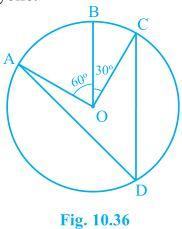Solution:
It is given that,
AOC = AOB + BOC
So, AOC = 60° + 30°
∴ AOC = 90°
It is known that an angle which is subtended by an arc at the centre of the circle is double the angle subtended by that arc at any point on the remaining part of the circle.
So,
= (½) × 90° = 45°.

Q.2. A chord of a circle is equal to the radius of the circle. Find the angle subtended by the chord at a point on the minor arc and also at a point on the major arc.
Solution: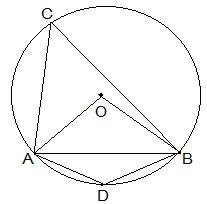Here, the chord AB is equal to the radius of the circle. In the above diagram, OA and OB are the two radii of the circle.
Now, consider the ΔOAB. Here,
AB = OA = OB = radius of the circle.
So, it can be said that ΔOAB has all equal sides and thus, it is an equilateral triangle.
∴ AOC = 60°
And, ACB = ½ AOB So, ACB = ½ × 60° = 30°
Now, since ACBD is a cyclic quadrilateral,
ADB + ACB = 180° (Since they are the opposite angles of a cyclic quadrilateral)
So, ADB = 180° - 30° = 150°
So, the angle subtended by the chord at a point on the minor arc and also at a point on the major arc are 150° and 30° respectively.

Q.3. In Fig. 10.37, PQR = 100°, where P, Q and R are points on a circle with centre O. Find OPR.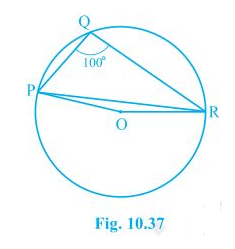Solution:
Since angle which is subtended by an arc at the centre of the circle is double the angle subtended by that arc at any point on the remaining part of the circle.
So, the reflex POR = 2 × PQR
We know the values of angle PQR as 100°
So, POR = 2 × 100° = 200°
∴ POR = 360° - 200° = 160°
Now, in ΔOPR,
OP and OR are the radii of the circle
So, OP = OR
Also, OPR = ORP
Now, we know sum of the angles in a triangle is equal to 180 degrees
So,
POR + OPR + ORP = 180°
OPR + OPR = 180° - 160°
As OPR = ORP
2OPR = 20°
Thus, OPR = 10°.

Q.4. In Fig. 10.38, ABC = 69°, ACB = 31°, find BDC.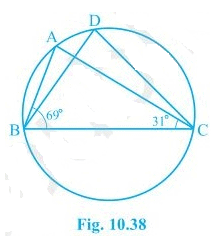Solution:
We know that angles in the segment of the circle are equal so,
∠BAC = ∠BDC
Now in the in ΔABC, the sum of all the interior angles will be 180°
So, ∠ABC + ∠BAC + ∠ACB = 180°
Now, by putting the values,
∠BAC = 180° - 69° - 31°
So, ∠BAC = 80°
∴ ∠BDC = 80°.

Q.5. In Fig. 10.39, A, B, C and D are four points on a circle. AC and BD intersect at a point E such that ∠ BEC = 130° and ∠ ECD = 20°. Find BAC.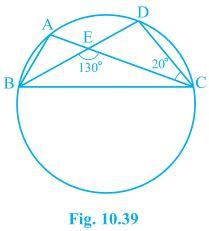Solution:
We know that the angles in the segment of the circle are equal.
So,
∠ BAC = ∠ CDE
Now, by using the exterior angles property of the triangle
In ΔCDE we get,
∠ CEB = ∠ CDE + ∠ DCE
We know that ∠ DCE is equal to 20°
So, ∠ CDE = 110°
∠ BAC and ∠ CDE are equal
∴ ∠ BAC = 110°.

Q.6. ABCD is a cyclic quadrilateral whose diagonals intersect at a point E. If ∠ DBC = 70°, ∠ BAC is 30°, find ∠ BCD. Further, if AB = BC, find ∠ ECD.
Solution:
Consider the following diagram.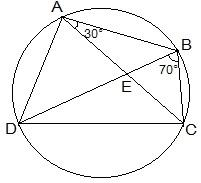Consider the chord CD,
We know that angles in the same segment are equal.
So, ∠ CBD = ∠ CAD
Now, ∠ BAD will be equal to the sum of angles BAC and CAD.
= 30° + 70°
We know that the opposite angles of a cyclic quadrilateral sums up to 180 degrees.
So,
∠ BCD + ∠ BAD = 180°
It is known that ∠ BAD = 100°
So, ∠ BCD = 80°
Now consider the ΔABC.
Here, it is given that AB = BC
Also, ∠ BCA = ∠ CAB (They are the angles opposite to equal sides of a triangle)
∠ BCA = 30°
also, ∠ BCD = 80°
∠ BCA + ∠ ACD = 80°
Thus, ∠ ACD = 50° and ∠ ECD = 50°.

Q.7. If diagonals of a cyclic quadrilateral are diameters of the circle through the vertices of the quadrilateral, prove that it is a rectangle.
Solution:
Draw a cyclic quadrilateral ABCD inside a circle with center O such that its diagonal AC and BD are two diameters of the circle.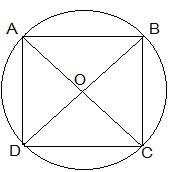We know that the angles in the semi-circle are equal.
So, ∠ ABC = ∠ BCD = ∠ CDA = ∠ DAB = 90°
So, as each internal angle is 90°, it can be said that the quadrilateral ABCD is a rectangle.

Q.8. If the non-parallel sides of a trapezium are equal, prove that it is cyclic.
Solution:
Construction:
Consider a trapezium ABCD with AB ║CD and BC = AD.
Draw AM, CD and BN, CD.
In ΔAMD and ΔBNC,
The diagram will look as follows: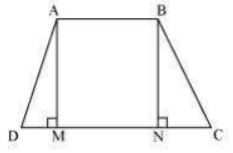In ΔAMD and ΔBNC,
AMD = BCN (By construction, each is 90º)
AM = BN (Perpendicular distance between two parallel lines is same)
ΔAMD, ΔBNC (RHS congruence rule)
BAD and BCD = 180º [Using equation (1)]
This equation shows that the opposite angle are supplementary.
Therefore, ABCD is a cyclic quadrilateral.

Q.9. Two circles intersect at two points B and C. Through B, two line segments ABD and PBQ are drawn to intersect the circles at A, D and P, Q respectively (see Fig. 10.40). Prove that ∠ ACP = ∠ QCD.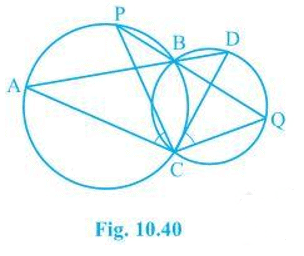Solution: Construction:
Join the chords AP and DQ.
For chord AP, we know that angles in the same segment are equal.
So, ∠ PBA = ∠ ACP ......(i)
Similarly for chord DQ,
∠ DBQ = ∠ QCD .....(ii)
It is known that ABD and PBQ are two line segments which are intersecting at B.
At B, the vertically opposite angles will be equal.
∴ ∠ PBA = ∠ DBQ ......(iii)
From equation (i), equation (ii) and equation (iii) we get,
∠ ACP = ∠ QCD.

Q.10. If circles are drawn taking two sides of a triangle as diameters, prove that the point of intersection of these circles lie on the third side.
Solution:
First draw a triangle ABC and then two circles having diameter as AB and AC respectively.
We will have to now prove that D lies on BC and BDC is a straight line.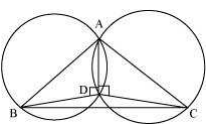Proof:
We know that angle in the semi-circle are equal
∴ ∠ BDC is straight line.
So, it can be said that D lies on the line BC.

Q.11. ABC and ADC are two right triangles with common hypotenuse AC. Prove that ∠ CAD = ∠CBD.
Solution:
We know that AC is the common hypotenuse and ∠ B = ∠ D = 90°.
Now, it has to be proven that ∠ CAD = ∠ CBD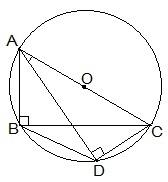Since, ∠ ABC and ∠ ADC are 90°, it can be said that They lie in the semi-circle.
So, triangles ABC and ADC are in the semi-circle and the points A, B, C and D are concyclic.
Hence, CD is the chord of the circle with center O.
We know that the angles which are in the same segment of the circle are equal.
∴ ∠ CAD = ∠ CBD

Q.12. Prove that a cyclic parallelogram is a rectangle.
Solution:
It is given that ABCD is a cyclic parallelogram and we will have to prove that ABCD is a rectangle.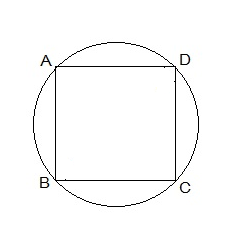Proof:
Let ABCD be a cyclic parallelogram.
A + C = 180º (Opposite angles of a cyclic quadrilateral)....(1)
We know that opposite angles of a parallelogram are equal.
A = C and B = D
From equation (1),
A + C = 180º
A + A = 180º
2A = 180º
Parallelogram ABCD has one of its interior angles as 90º.
Thus, ABCD is a rectangle.

The document Ex 10.5 NCERT Solutions- Circles Notes | Study Mathematics (Maths) Class 9 - Class 9 is a part of the Class 9 Course Mathematics (Maths) Class 9.
All you need of Class 9 at this link: Class 9Use Code STAYHOME200 and get INR 200 additional OFF Use Coupon Code

Top Courses for Class 9Mathematics (Maths) Class 9

46 videos|351 docs|110 tests

Top Courses for Class 9Track your progress, build streaks, highlight & save important lessons and more!

,

,

,

,

,

,

,

,

,

,

,

,

,

,

,

,

,

,

,

,

,

;